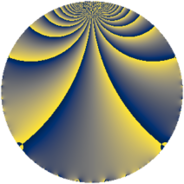# Properties

 Label 152.1.kLevel $152$ Weight $1$ Character orbit 152.k Rep. character $\chi_{152}(11,\cdot)$ Character field $\Q(\zeta_{6})$ Dimension $2$ Newform subspaces $1$ Sturm bound $20$ Trace bound $0$

# Related objects

## Defining parameters

 Level: $$N$$ $$=$$ $$152 = 2^{3} \cdot 19$$ Weight: $$k$$ $$=$$ $$1$$ Character orbit: $$[\chi]$$ $$=$$ 152.k (of order $$6$$ and degree $$2$$) Character conductor: $$\operatorname{cond}(\chi)$$ $$=$$ $$152$$ Character field: $$\Q(\zeta_{6})$$ Newform subspaces: $$1$$ Sturm bound: $$20$$ Trace bound: $$0$$

## Dimensions

The following table gives the dimensions of various subspaces of $$M_{1}(152, [\chi])$$.

Total New Old
Modular forms 6 6 0
Cusp forms 2 2 0
Eisenstein series 4 4 0

The following table gives the dimensions of subspaces with specified projective image type.

$$D_n$$ $$A_4$$ $$S_4$$ $$A_5$$
Dimension 2 0 0 0

## Trace form

 $$2q - q^{2} + q^{3} - q^{4} + q^{6} + 2q^{8} + O(q^{10})$$ $$2q - q^{2} + q^{3} - q^{4} + q^{6} + 2q^{8} - 2q^{11} - 2q^{12} - q^{16} - 2q^{17} - q^{19} + q^{22} + q^{24} - q^{25} + 2q^{27} - q^{32} - q^{33} - 2q^{34} + 2q^{38} + q^{41} - 2q^{43} + q^{44} + q^{48} + 2q^{49} + 2q^{50} + 2q^{51} - q^{54} - 2q^{57} + q^{59} + 2q^{64} - q^{66} + q^{67} + 4q^{68} + q^{73} - 2q^{75} - q^{76} + q^{81} + q^{82} - 2q^{83} - 2q^{86} - 2q^{88} - 2q^{89} - 2q^{96} + q^{97} - q^{98} + O(q^{100})$$

## Decomposition of $$S_{1}^{\mathrm{new}}(152, [\chi])$$ into newform subspaces

Label Dim. $$A$$ Field Image CM RM Traces $q$-expansion
$$a_2$$ $$a_3$$ $$a_5$$ $$a_7$$
152.1.k.a $$2$$ $$0.076$$ $$\Q(\sqrt{-3})$$ $$D_{3}$$ $$\Q(\sqrt{-2})$$ None $$-1$$ $$1$$ $$0$$ $$0$$ $$q+\zeta_{6}^{2}q^{2}-\zeta_{6}^{2}q^{3}-\zeta_{6}q^{4}+\zeta_{6}q^{6}+\cdots$$